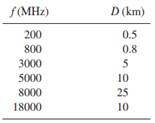Create an Account

Home / Questions / 1 A microwave receiver has a total input noise power of 102 dBm and an overall noise figur...

1 A microwave receiver has a total input noise power of 102 dBm and an overall noise figure of 4 dB For a minimum C N ratio of 20 dB at the input to the FM detector determine the minimum receive

1. A microwave receiver has a total input noise power of -102 dBm and an overall noise figure of 4 dB. For a minimum C/N ratio of 20 dB at the input to the FM detector, determine the minimum receive carrier power.

2. Determine the path loss for the following frequencies and distances:3. Determine the fade margin for a 30-km microwave hop. The RF frequency is 4 GHz, the terrain is water, and the reliability objective is 99.995%.

Jul 28 2020 View more View LessGet Solution